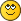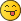Scaling the matrix - Printable Version +- LDraw.org Discussion Forums (https://forums.ldraw.org) +-- Forum: LDraw Programs (https://forums.ldraw.org/forum-7.html) +--- Forum: LDraw Editors and Viewers (https://forums.ldraw.org/forum-11.html) +--- Thread: Scaling the matrix (/thread-23734.html) Pages: 1 2 3 RE: Scaling the matrix - N. W. Perry - 2019-11-08 (2019-11-06, 9:33)Owen Dive Wrote: No - at least, not necessarily. Rotate a part about some arbitrary axis and you'll see all 9 numbers in the matrix change. I don't remember what the mathematical terminology is (and I may even get some of this completely wrong!) but it goes something like this: Imagine you have a part and you want to describe its position and orientation relative to some coordinate system. Position is easy, you say "the centre of the part is at so many units along the x axis, so many along the y axis, and so many along the z axis". But orientation is not so clear. You have to say something like "If I start at the centre of the part, and go forward one unit relative to the part, I'll end up at position x1, y1, z1, and if I go left one unit relative to the part, I end up at position x2, y2, z2, and if I go up one unit, I end up at x3, y3, z3". The 9 numbers in the matrix are the coordinates of these three points. Now suppose I want to scale in the (say) front/back axis, by a factor of 2. I can start at the centre of the part and move _2_ units forward. I'll end up at position 2*x1, 2*y1, 2*z1. If I put those numbers in my matrix, the rendering engine will assume that those coordinates were generated at 1 unit distance, and draw all the pixels two times further forward than before. Does that make it any clearer? Or have I just made it more confusing? Edit: Actually, on re-reading this thread, I suspect that you probably understand all that. If I could delete my post, I would. Yeah, I guess what I was asking was more like what each separate box can be said to represent. The boxes themselves aren't complete coordinates, but factors—amounts of change, I guess? And you're right, when you combine transformations (such as rotating around multiple axes), you'll end up populating more boxes than with a single operation (and combining the values of some boxes together). But is there a simply way to say, for example, the top-left box represents the ratio of an original x value to its new x position, and so forth? RE: Scaling the matrix - Travis Cobbs - 2019-11-08 (2019-11-08, 15:35)N. W. Perry Wrote: Yeah, I guess what I was asking was more like what each separate box can be said to represent. The boxes themselves aren't complete coordinates, but factors—amounts of change, I guess? And you're right, when you combine transformations (such as rotating around multiple axes), you'll end up populating more boxes than with a single operation (and combining the values of some boxes together). But is there a simply way to say, for example, the top-left box represents the ratio of an original x value to its new x position, and so forth? The short answer is that it's complicated. Standard 3D transformations (rotating, scaling, and translating) each have a specific set of values that they operate on in a standard 4x4 3D transformation matrix. (For references, the 3x3 matrix you see is the upper left corner of a 4x4 general transformation matrix.) Things are complicated by the fact that there are actually two different ways that people apply the transformations, and the ways are mirrored across the matrix's top-left to bottom-right diagonal. So, in one way, the top three elements of the 4th column represent the x,y,z translation, and in the mirrored way, the left three elements of the 4th row represent the same thing. Both ways produce the same end results, as long as they are used consistently. Here is a tutorial that lists how the typical transformations are applied to the standard matrix. It uses the representation that has the x,y,z translation as the left three elements of the 4th column. I think that most LDraw software uses the top three elements of the 4th row for the x,y,z translation, in which case you would need to mirror all the sample matrices along the top-left to bottom-right diagonal. I could be misremembering; it has been a long time since I paid any attention to LDView's matrix math. Also, LDCad presents the x,y,z translation as a separate set of fields, and doesn't provide access to the remaining 4 fields in the 4x4 matrix. If you look at the tutorial, you will see that these 4 values are always 0,0,0,1, so that doesn't really matter. But if you look specifically at the scale transformation, you will see exactly what Philo said originally: the three fields along the diagonal represent the x, y, and z scale factors. (Remember that in LDraw, "up" is -Y.) RE: Scaling the matrix - Roland Melkert - 2019-11-08 (2019-11-08, 21:11)Travis Cobbs Wrote: But if you look specifically at the scale transformation, you will see exactly what Philo said originally: the three fields along the diagonal represent the x, y, and z scale factors. (Remember that in LDraw, "up" is -Y.) Maybe it's me but I get the feeling people are over complicating this. The columns in the LDCad dialog indicate the direction of the part's local xyz axis' as seen from absolute space. First column indicates the vector of the X-ax's (1,0,0 as it's non rotated). 2nd the Y-axis and 3rd the z-axis. Normally all these vectors are normalized (length == 1.0), with any other length scaling will be applied. You could also read it from a row's perspective to go the other way (direction of world axis' as seen from local part space) RE: Scaling the matrix - N. W. Perry - 2019-11-09 (2019-11-08, 21:34)Roland Melkert Wrote: Maybe it's me but I get the feeling people are over complicating this. The columns in the LDCad dialog indicate the direction of the part's local xyz axis' as seen from absolute space. First column indicates the vector of the X-ax's (1,0,0 as it's non rotated). 2nd the Y-axis and 3rd the z-axis. Normally all these vectors are normalized (length == 1.0), with any other length scaling will be applied. You could also read it from a row's perspective to go the other way (direction of world axis' as seen from local part space) And now it makes sense.RE: Scaling the matrix - N. W. Perry - 2020-03-28 Same question, different parameters: what if I'm rotating an assembly, where the connection points are offset? In this case, the distance between the two pin holes needs to be stretched by a scale factor of 1.01242, but that axis isn't orthogonal to the parts themselves. How would I calculate the scale factor to stretch the assembly in the X direction?RE: Scaling the matrix - Roland Melkert - 2020-03-28 (2020-03-28, 2:04)N. W. Perry Wrote: gonal to the parts themselves. How would I calculate the scale factor to stretch the assembly in the X direction? 1. Put a temporary pin at the other end of the 1x8 brick to get a rotation angle using selection info. 2. Apply the rotation 3. Select the 1x8 brick and set relative gird ( o ) 4. Press enter to edit the reference. 5. Put  1.01242 in the top left matrix field. Do note it's not 'officially' supported to scale stuff at the model level in LDCad. Main thing to keep an eye on is to select something non scaled before adding new parts, or those will be scaled too. RE: Scaling the matrix - N. W. Perry - 2020-03-28 Mm…I couldn't get that method to compute, for some reason. What did work, though, was to apply the scale factor to both the X and Y directions of the assembly. That makes sense, because… The rotation point and the connection point (the hole in the 1x2 brick) form the hypotenuse of a right triangle. Its length is ~126.49, and I need to stretch it to ~128.06, giving the scale factor of 1.01242. Since the ratio of the sides of a triangle remain the same as long as the angles don't change, then if I want to transform my assembly in the X direction using that same scale factor, I have to apply it in Y as well, to keep the proportions equal. So after entering 1.01242 into both the X and Y boxes, my hypotenuse now measures 128.06, and I can just apply the rotation shown (32.91°), because again, the angles in my triangle stay the same. Now, what if I want to stretch my assembly only in the X direction, as originally planned? (Don't ask why—I guess I figured the distortion would be less visually apparent that way.) Well, I know I want my hypotenuse to be 128.06, and since I'm not changing the Y dimension, I know that the short side of my triangle remains at 40 (the vertical distance between the holes in the 1x2 and 1x8 bricks). And since it's a right triangle, I know I have an angle of 90°—and knowing 2 sides plus 1 angle is enough to calculate a whole triangle! I can either algebraically solve the Pythagorean theorem for b, or just plug the values into my favorite online triangle calculator.Turns out, my third side needs to be 121.6625; it's currently 120, so that's a scale factor of 1.01379. Enter that into the X matrix, use Selection Info to get the new rotation value (because I changed the ratio of my triangle sides, therefore the angles changed as well), apply that to the assembly—now it fits!Sorry to ask my question, only to answer myself right away!I was actually stumped, but for some reason posting these puzzlers here helps me think them through…and maybe will help someone else later on. And it's worth noting that, as usual, my answer lies in the fact that you can solve any triangle if you know at least one side, plus any other 2 of the sides or angles. RE: Scaling the matrix - Roland Melkert - 2020-03-28 (2020-03-28, 5:08)N. W. Perry Wrote: Mm…I couldn't get that method to compute, for some reason. I shouldn't answer posts that late, I thought the 1x8 brick was supposed to go between the two pinsYou can still use the relative grid method though, just select all red parts before scaling. RE: Scaling the matrix - N. W. Perry - 2020-03-28 (2020-03-28, 19:21)Roland Melkert Wrote: I shouldn't answer posts that late, I thought the 1x8 brick was supposed to go between the two pinsYou can still use the relative grid method though, just select all red parts before scaling. Yes!Relative scaling does still work—I tried it both as a submodel and as a group, but is there anything I need to be careful of? Sometimes I find that I have to re-snap the parts of a group after scaling, but that may be only when I'm using the absolute grid. (Also, it appears that scaling will account for the origin of a submodel, but not the custom center of a group.) RE: Scaling the matrix - Roland Melkert - 2020-03-28 (2020-03-28, 20:53)N. W. Perry Wrote: (Also, it appears that scaling will account for the origin of a submodel, but not the custom center of a group.) In LDCad scaling at the model level is basically misusing rotations. The whole internals 'assume' all rotations are non scalar. Using it couldn't do any real harm but it was never designed for it.